# Bond Energies James Bond Energies What Is Bond

• Slides: 17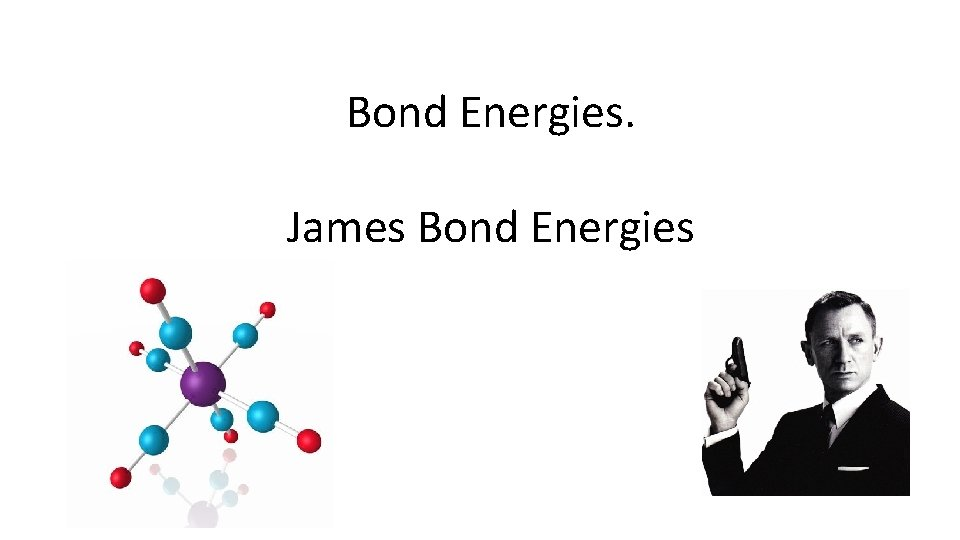Bond Energies. James Bond EnergiesWhat Is Bond Energy? Think of a bond like a spring holding two atoms together: If you pull them apart a little bit, they will spring back together If you squish them together a little bit, they will push back apart There is a natural length to the spring (or bond) that it wants to be. BUT! If you pull them hard enough apart, you can break the spring. Bond energy is how much energy you have to use to pull the atoms apart and break the bond.Bond Energy In Graph Form This is called the Lennard-Jones potential (you don’t need to know the name): (The values on the y-axis are somewhat arbitrary— only the difference between them matters) The atoms want to be 74 pm apart—at the bottom of the hill. This is the bond length. If we push them closer together (point 4) or pull them farther apart (point 2), they will spring back to 74 pm apart (point 3). But if we put 432 k. J/mol in, we can pull them all the way to point 1 and they are no longer attached to each other. This value is the bond energy.Bond Energy In Graph Form Some bonds are shorter/longer, and some are weaker/stronger The X 2 bond is very short but not very strong: it is two very small atoms that can get close together, but only make one bond (like H 2) The Z 2 bond is much longer, but not much stronger. This would be larger atoms making one bond (like Cl 2), or maybe making an unusually weak double bond (like O 2) Y 2 is shorter than Z 2 and very strong, so this is either a very strong double bond, or a triple bond (like N 2).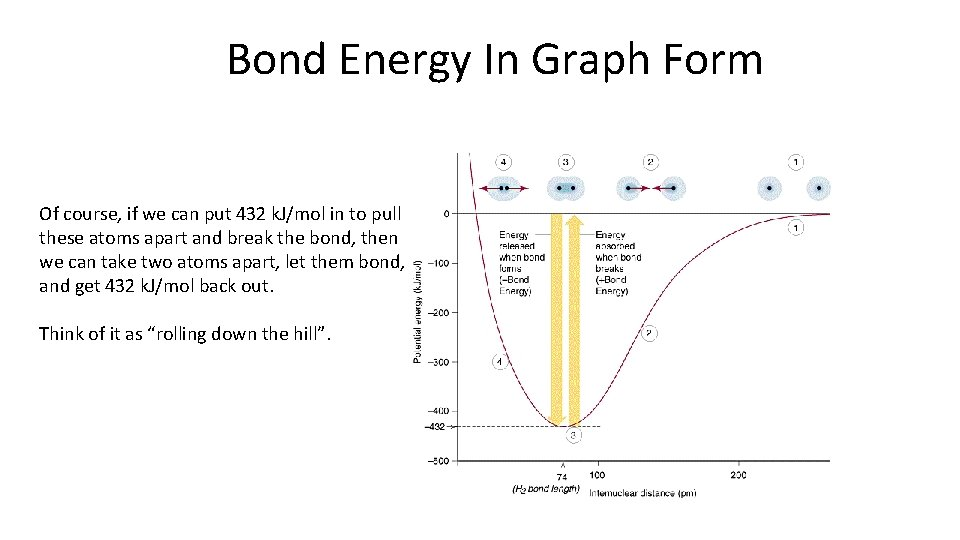Bond Energy In Graph Form Of course, if we can put 432 k. J/mol in to pull these atoms apart and break the bond, then we can take two atoms apart, let them bond, and get 432 k. J/mol back out. Think of it as “rolling down the hill”.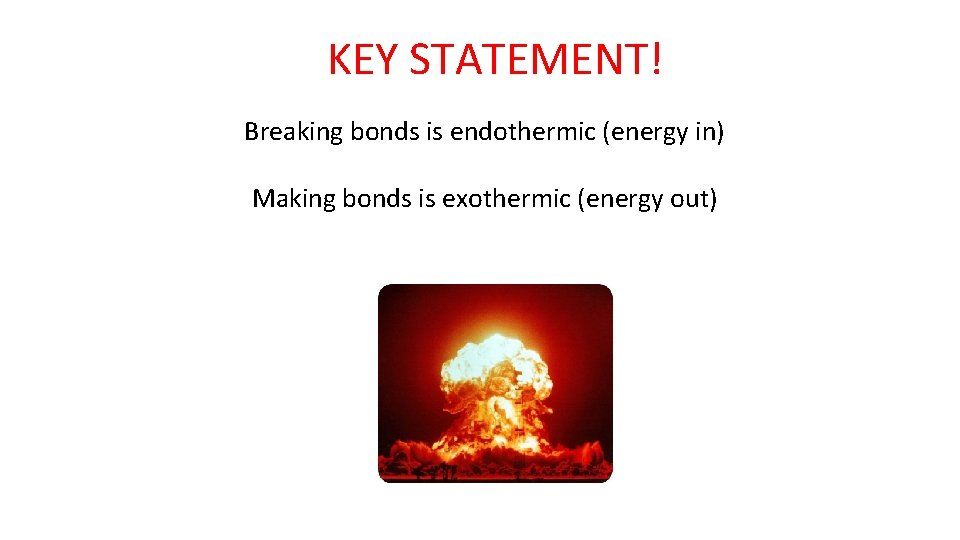KEY STATEMENT! Breaking bonds is endothermic (energy in) Making bonds is exothermic (energy out)Using Bond Energies What’s the point? If we have a reaction that uses and makes only covalent compounds, we can find DH using bond energies. Conveniently, people have put together huge tables showing the bond energies for all kinds of different bonds (these are always given as positive—breaking the bond)Using Bond Energies What’s the point? If we have a reaction that uses and makes only covalent compounds, we can find DH using bond energies. Conveniently, people have put together huge tables showing the bond energies for all kinds of different bonds (these are always given as positive—breaking the bond) Less conveniently, these values are only general estimations—not every single Carbon —Hydrogen bond in the world is the same as all the others. Our DH will be an approximation.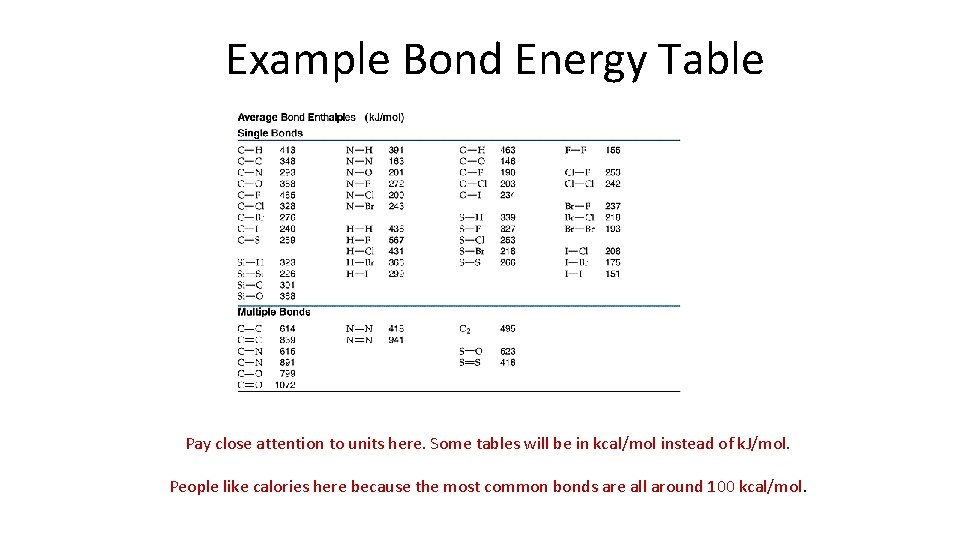Example Bond Energy Table Pay close attention to units here. Some tables will be in kcal/mol instead of k. J/mol. People like calories here because the most common bonds are all around 100 kcal/mol.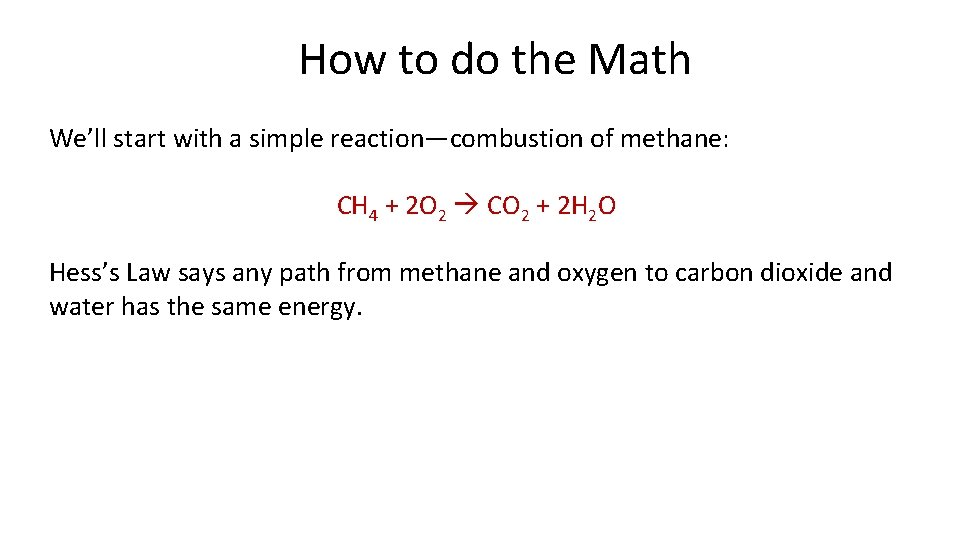How to do the Math We’ll start with a simple reaction—combustion of methane: CH 4 + 2 O 2 CO 2 + 2 H 2 O Hess’s Law says any path from methane and oxygen to carbon dioxide and water has the same energy.CH 4 + 2 O 2 CO 2 + 2 H 2 O Breaking all the reactants apart: We broke (values from earlier table): 4 C-H bonds: 413 k. J/mol each * 4 = 1652 k. J/mol 2 O=O bonds: 495 k. J/mol each * 2 = 990 k. J/mol (making sure to use the double bond value) So in total we had to put in 2642 k. J/mol to break the atoms apart.CH 4 + 2 O 2 CO 2 + 2 H 2 O Putting the products together We made (values again from earlier table. Negative because we’re making bonds): 4 O-H bonds: -463 k. J/mol each * 4 = -1852 k. J/mol 2 C=O bonds: -799 k. J/mol each * 2 = -1598 k. J/mol (making sure to use the double bond value) So in total we had got out -3450 k. J/mol by putting atoms together.CH 4 + 2 O 2 CO 2 + 2 H 2 O All together now: 2642 k. J/mol put in to break the bonds -3450 k. J/mol out by making the new bonds So overall our process has a DH = -808 k. J/mol and is exothermic.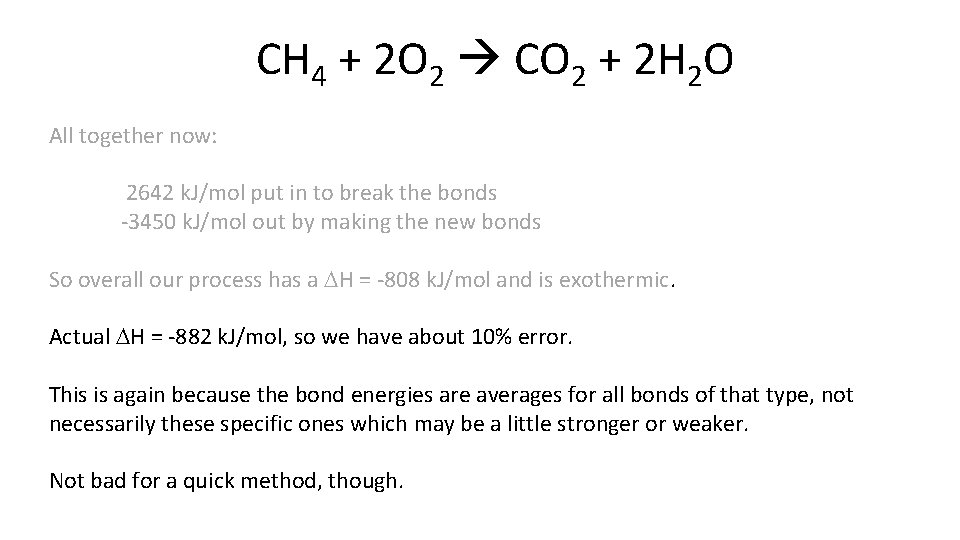CH 4 + 2 O 2 CO 2 + 2 H 2 O All together now: 2642 k. J/mol put in to break the bonds -3450 k. J/mol out by making the new bonds So overall our process has a DH = -808 k. J/mol and is exothermic. Actual DH = -882 k. J/mol, so we have about 10% error. This is again because the bond energies are averages for all bonds of that type, not necessarily these specific ones which may be a little stronger or weaker. Not bad for a quick method, though.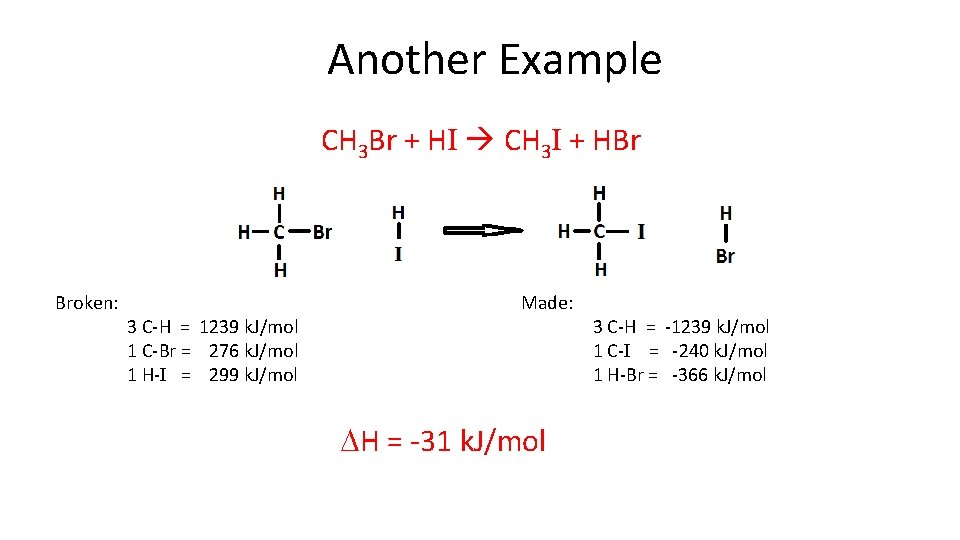Another Example CH 3 Br + HI CH 3 I + HBr Broken: 3 C-H = 1239 k. J/mol 1 C-Br = 276 k. J/mol 1 H-I = 299 k. J/mol Made: DH = -31 k. J/mol 3 C-H = -1239 k. J/mol 1 C-I = -240 k. J/mol 1 H-Br = -366 k. J/molAnother Example CH 3 Br + HI CH 3 I + HBr Broken: 1 C-Br = 276 k. J/mol 1 H-I = 299 k. J/mol Made: 1 C-I = -240 k. J/mol 1 H-Br = -366 k. J/mol It’s totally ok to recognize that nothing changes with the C-H bonds and just leave them off the lists. However, I find that people make more mistakes when they do this for complicated structures/reactions.Summary What do you need to know? • Breaking bonds is endothermic. Making bonds is exothermic. • How to recognize bond length and bond energy from the graphs. • How to compare two bonding graphs. • Be able to use bond energies to approximate DH.